/
Features

# Exploring Spring Model

The first time Gustavo Oliveira implemented a spring model, it fascinated him for hours. The effect itself is amazingly realistic, and its implementation is fairly simple. This article reviews the implementation of a spring model from its simplest form to more sophisticated applications, taking the subject a step beyond the material available in most references.

The first time I implemented a spring model, it fascinated me for hours. The effect itself is amazingly realistic, and its implementation is fairly simple. Fortunately, I found a lot of articles, references, and source code to help with my research. Nevertheless, as I went further down the road, I noticed that in most cases these references limited to the standard applications of spring models -- string, cloth and jelly.

This article reviews the implementation of a spring model from its simplest form to more sophisticated applications, taking the subject a step beyond the material available in most references.

Spring Basics

Before modeling springs with the computer, you need to understand the basic principles of springs from your classic physics book.

As you compress or extend a spring, it creates a force that is opposed to direction fo the force that you are applying. This force is mathematically equated by the formula:

F = -k*Dx
Dx = xc-xi

Where F is the resultant force, k is the spring coefficient, and Dx the distance from its inertial position (xc = current distance, and xi = distance at the inertial position).

Mathematically, you can think of a spring as being two points separated from a distance x. This is its inertial position. In other words, if you don't move these points, no force will be generated because Dx = 0. If you try to compress the distance between these points the Dx will be negative, generating a positive force (expansion). If you try to separate these points the Dx will be positive, generating a negative force (compression). The picture below illustrates these cases.

Pa -/\/\/\/\/\/\/\/\/\/\/\/\/\/\/\/\/\/\/\/\/\/\- Pb (Dx = 0, F = 0)
Pa -/\/\/\/\/\/\/\/\/\/\/\/\/\/\/\/\- Pb (Dx < 0, F > 0)
Pa -/\/\/\/\/\/\/\/\/\/\/\/\/\/\/\/\/\/\/\/\/\/\/\/\/\/\/\- Pb (Dx > 0, F < 0)

Springs (using "ascii art")

The k coefficient is called the elasticity coefficient, and weights the spring's final force. In other words, a spring with bigger k is stiffer because it creates a larger force from its inertial state. Conversely, a spring with a smaller k is more flexible because it creates a smaller force from its inertial state.

Spring Force Implementation

Implement the spring force is a direct application of the first two equations. In other words, first compute the distance of the spring's extremities (Pa and Pb). This is done through Pythagoras' theorem. Once you have the distance, you subtract from the inertial distance getting the Dx. Then, multiply this scalar value to the k coefficient and finally use the inverse of this value to compute the force.

As simple as it sounds, the implementation in C code has its details. First, the distance Dx, and the k coefficient are scalars, but the force itself is a vector. Therefore, it requires a direction, which can be from Pa to Pb, or from Pb to Pb. As of right now, the code below always use the direction Pb to Pa, or mathematically Fdir = Pb-Pa.

As the force's direction is normalized, you can use the distance you already computed for the Dx for the normalization. You must be careful is to avoid division by zero when normalizing the vector. This is a nasty condition and it usually does not happen, but you have to do something about it in case it does. You have two options in this particular case: assign a random force to the points, or null out the force. Theoretically, the force should be big enough that as the points get closer they will never occupy the same space. Therefore, it seems to be a good option to randomly assign a massive force in this case. However, this can cause undesirable artifacts, because some points inside the spring model might fly a part with extreme velocity when compared with others. Although nulling out seems wrong, it doesn't cause any major problems and preserves the spring's stability. The code below shows the implementation; it's a little redundant for sake of clarity. I null out the force in case of potential division by zero.

Listing 1: Computing A Spring's Force

#define SPRING_TOLERANCE (0.0000000005f)

void SpringComputeSingleForce (VECTOR *force, VECTOR *pa, VECTOR *pb, float k, float delta_distance)
{
VECTOR force_dir;
float intensity;
float distance;
float delta;

//get the distance
float dx = pb->x-pa->x;
float dy = pb->y-pa->y;
float dz = pb->z-pa->z;

distance = (float) sqrt ((dx*dx)+(dy*dy)+(dz*dz));

if (distance < SPRING_TOLERANCE)
{
force->x =
force->y =
force->z = 0.0f;

return;
}

force_dir.x = dx;
force_dir.y = dy;
force_dir.z = dz;

//normalize
force_dir.x /= distance;
force_dir.y /= distance;
force_dir.z /= distance;

//force
delta = distance-delta_distance;
intensity = k*delta;

//store
force->x = force_dir.x*intensity;
force->y = force_dir.y*intensity;
force->z = force_dir.z*intensity;
}

Connecting Springs

Computing the spring force in one direction for a single spring doesn't do much. The power of springs comes when you connect them into a network. Then, the computed force of one spring affects the entire network. In other words, if one point moves, it creates a force that propagates through the connected springs. This results into a realistic movement of connected points simulating what would happen in real life.

The three common applications of connected springs are string, cloth, and jelly. These models are very similar. The only difference is their initial geometric arrangement and how their springs are connected. For instance, to model a string you connect springs as a line (one dimension). To model cloth you connect springs as a plane (two dimensions). Finally, to model jelly you connect springs as a volume (three dimensions).

Before implementing these applications, take a closer look of how two springs can be connected. Figure 1 shows two springs represented by two think colored lines, with the extremities represented by three points. The forces that can be created between these points are shown with arrows.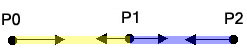Figure 1: Forces between Two Springs

From Figure1, two connected springs can generate four forces. For example, if you move and hold P0 the left, the force P0->P1 will be created to pull P0 back closer to P1. Also, the force P1->P0 will be created to move P1 closer to P0. As P1 is also connected to P2, the forces P1->P2 and P2->P1 will be also created to approximate these points. If you had more points, the forces will keep propagating through the springs because of its connections.

You don't have to necessarily follow the arrangement of Figure 1. For example Figure 2 shows four springs connected together in a different manner. Again, the thick colored lines represent the springs, the points its extremities, and the arrows the forces that can be created between them.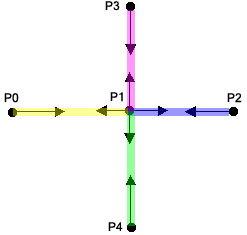Figure 2: Forces between Four Springs

In this arrangement there are four possible acting forces on P1 derived from P0, P2, P3 and P4. Also, you don't necessarily have to connect a point to its neighbors as in the previous figures. For instance you can add another spring connecting P0 to P2. Or even add more than one spring connecting two points. In fact, they way you build your spring network is entirely up to you. The arrangement you choose, however, will affect your final spring model behavior.

Based on this idea, the figures below show ways to connect springs for a string, a cloth and a jelly respectively. In each arrangement the edges represents one spring. The blue dots are the extremities of the springs.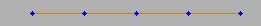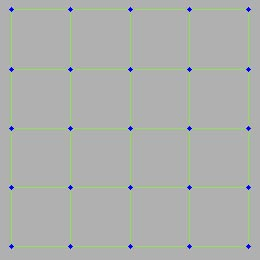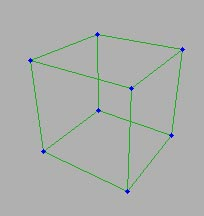Figures 3-5: String Arrangement, Cloth Arrangement, Jelly Arrangement*

In each configuration, if you move one point it will realistically simulate the object's behavior. However, this is not entirely true for the particular jelly arrangement presented. Indeed the arrangement of Figure 5 is unstable. If you model a jelly exactly like in Figure 5, moving one of its points will morph the jelly into a different object. Soon the original cubical shape will be be gone. Why? Because the spring force formula requires the scalar Dx, and its direction is irrelevant. Therefore, if you simply rotate two connected springs, they can move to another configuration where no forces will be created. Figure 6 and Figure 7 below clarify the last statement by showing two sets of springs that do not create any forces. In both cases the Dx = 0, but their geometric shape are different.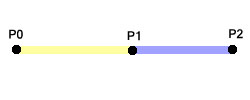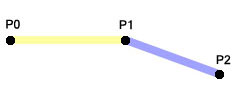Figures 6-7: Inertial Springs (Dx = 0), Inertial Springs (Dx = 0)

Because the springs can rotate around their extremities without creating any forces, you must be careful when modeling and connecting your springs. This is probably the most important part of the implementation. You must design your spring model to be able to swing nicely without morphing it into something unrecognizable. Also, your design should use the least number of springs for the sake of calculation time. Figure 8 composes a stable arrangement for a jelly model. In this arrangement there are a lot more springs than the previous one, and some vertices connect up to six springs. This is still not the only way of connecting them to create a stable model. For example you can stack the cloth model and connect all the planes with more springs. This configuration will be computationally more intense, and it will behave differently than the arrangement of Figure 8. Again, the final arrangement is up to you, and it depends on what you are trying to simulate.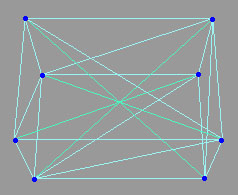Figure 8: Stable Arrangement For Jelly

Implementing a String

With the basic information already presented, let's look in how to implement the simplest spring model --a string. Despite of its simplicity, once you understand how to implement a string, other models become just an extension to the same basic idea.

To begin, you need a data structure that allows you to save the spring's position, compute its forces, and enable connection to other springs. Therefore, it is easier to design your data for the spring's extremities instead of each spring itself. In this implementation, each extremity of a spring is called a spring node. Two spring nodes compose one spring. As a polygonal mesh has a list of vertices, the spring model will have a list of spring nodes. The code below shows the basic data structure for each spring node, and the helper data structure VECTOR.

Listing 2: Spring Node Data Structure

#define SPRING_MAX_NEIGHBOR_COUNT (128)

typedef struct _vector
{
float x;
float y;
float z;

} VECTOR;

typedef struct _spring_node
{
VECTOR pos; //current position
VECTOR vel; //current velocity
VECTOR force; //resultant

float mass; //nodes's mass

SPRING_NODE* neighbor_node[SPRING_MAX_NEIGHBOR_COUNT];
float neighbor_x[SPRING_MAX_NEIGHBOR_COUNT]; //distances
int neighbor_count;

} SPRING_NODE;

The spring node data structure above holds the current position, velocity, resultant force and pointers to the neighbor nodes. It also saves the distance x from the neighbor nodes at the inertial state -- necessary to compute the resultant force. Yet, Listing 2 is a little nasty. Like Listing 1, it was written that way simply for educational purposes. For instance, this data structure is used for build time only. Therefore, to save some memory, it would be better to use linked lists for the neighbor pointers and distances instead of arrays.

In a nutshell, the spring model is simply a list (array) of spring nodes. And to build a string (or any spring model) all you have to do is to set up the spring model initial geometric arrangement, and connect its nodes properly. Then you are ready to animate it, by computing the internal forces and applying to the points.

Before you see the animation loop details, take a closer look into the spring model data structure, followed by the string creation code. Listing 3 depicts the main data structures used to build any spring model. As you see it is nothing more than a collection of nodes, and extra fields for forces calculation, and debugging.

Listing 4 is the actual setup code for a string. It has four main parts. First, it allocates memory for the string data and assigns the main parameters to it. Second, it positions the nodes aligned to the xz positive axis. Third, connects the nodes and assigns the inertial distances for the nodes and its neighbors. The last part is where the spring model behavior is defined. This part of Listing 4 is fairly simple, and (hopefully!) easy to read. That's because it models a string, and the implementation takes advantage of the string's symmetry. The last part of Listing 4 is setting up the anchor point. This is a point that will not animate no matter how strong the forces are on it. The anchor points are used for the debugging and testing. To drag model around, you need at least point. In some models, as you will see later, the anchor points are useful to keep the spring model stable.

Listing 3: Spring Model Data Structure

#define SPRING_MAX_NODE_COUNT (4096)
#define SPRING_MAX_ANCHOR_NODE_COUNT (256)

typedef struct _spring_anchor
{
VECTOR pos;
SPRING_NODE* node;

} SPRING_ANCHOR;

typedef struct _spring
{
SPRING_NODE node[SPRING_MAX_NODE_COUNT]; //array of spring nodes
int node_count;

SPRING_ANCHOR anchor[SPRING_MAX_ANCHOR_NODE_COUNT]; //stability & debug
int anchor_count;

float k_coef;
float energy_loss;
float mass;

} SPRING;

Listing 4: Creating a String

SPRING* SpringCreateString (float distance, int num_nodes, float k_coef, float energy_loss, float mass)
{
SPRING* spring;

if ((distance < 0.005f) || (num_nodes < 3))
{
return (NULL);
}

/////////////////////////////////////////////////////////////
//allocate memory & setup parameters
/////////////////////////////////////////////////////////////

int mem_size;

mem_size = sizeof (SPRING);
spring = (SPRING*)MemoryAllocAndClear (mem_size); //allocate & zero out memory
if (!spring)
{
return (NULL);
}

spring->k_coef = k_coef;
spring->energy_loss = energy_loss;
spring->mass = mass;

//////////////////////////////////////////////////////////////////////
//setup nodes position aligned with the xz+ axis
//////////////////////////////////////////////////////////////////////

SPRING_NODE* node_curr;
int tmp0;
VECTOR pos_curr = {0.0f, 0.0f, 0.0f, 0.0f};

for (tmp0=0; tmp0
{
node_curr = &spring->node[tmp0];
node_curr->pos = pos_curr;
pos_curr.x += distance;
}

///////////////////////////////////////////////////////////////////////
//connect nodes and setup the inertial x distances
///////////////////////////////////////////////////////////////////////

//far left node
node_curr = &spring->node;
node_curr->neighbor_node = &spring->node;
node_curr->neighbor_x = distance;
node_curr->neighbor_count++;

//far right node
node_curr = &spring->node[num_nodes-1];
node_curr->neighbor_node = &spring->node[num_nodes-2];
node_curr->neighbor_x = distance;
node_curr->neighbor_count++;

//inner nodes
for (tmp0=1; tmp0
{
node_curr = &spring->node[tmp0];

//connect to the previous
node_curr->neighbor_node = &spring->node[tmp0-1];
node_curr->neighbor_x = distance;

//connect to the next
node_curr->neighbor_node = &spring->node[tmp0+1];
node_curr->neighbor_x = distance;

node_curr->neighbor_count += 2;
}

////////////////////////////////
// setup anchor node
////////////////////////////////

//far right node is the only anchor
spring->anchor.node = &spring->node;
spring->anchor.pos = spring->node.pos;

return (spring);
}

Once the geometry is ready and the nodes are connected, the next step is to implement the animation loop. Listing 5 illustrates the generic loop. Again, this code is redundant, and not optimized, for sake of clarity. The basic idea is to loop through all the nodes, compute all the forces on each node, and store the resultant force. Then, the resultant is used to add velocity to the node's position. Finally, the anchor points are "fixed" by not letting the forces act on them.

Listing 5: Animation Loop

void SpringComputeForces (SPRING* spring)
{
SpringAccumulateForces (spring);
SpringApplyForces (spring);
SpringAnchorCheck (spring);
}

To compute the final resultant force on each node, the neighbor nodes come into play. In Listing 6 for each node you compute the forces that all neighbor nodes apply to it. Then, you accumulate (add) all these forces and store it in the data structure. Do you remember in Listing 1 when only one direction was necessary to compute the force? This is because as you are looping through all nodes, and looping through all its neighbors as well. Therefore, at the end you will be computing both directions of the same force. In other words, when P0 is the current node, the force P1->P0 is computed. Then, when the current node is P1, the force P0->P1 is computed. As you are computing the same force (with opposite signs) twice, this can be optimized later. As of right now, to keep the loop easier to read, the code does it the slow way.

Listing 6: Accumulating the Forces on Each Spring Node

void SpringAccumulateForces (SPRING* spring)
{
int tmp1;
int tmp0;
VECTOR force_tmp;
SPRING_NODE* node_curr;
VECTOR* source;
VECTOR* dest;
float distance;
VECTOR resultant;

//accumulate all forces
for (tmp1=0; tmp1node_count; tmp1++)
{
node_curr = &spring->node[tmp1];

//null out resultant
resultant.x =
resultant.y =
resultant.z = 0.0f;

source = &node_curr->pos;

for (tmp0=0; tmp0neighbor_count; tmp0++)
{
dest = &node_curr->neighbor_node[tmp0]->pos;
distance = node_curr->neighbor_x[tmp0];

SpringComputeSingleForce (&force_tmp,
source,
dest,
spring->k_coef,
distance);

}

node_curr->force = resultant;
}
}

To finally animate the spring model you simply apply the resultant force of each to node to itself, making them to move. From your physics book, force and acceleration are related as following.

F = M*Acc
Acc = F/M

As the first equation shows, to compute the node's acceleration you divide the resultant force by the spring node's mass. If the force is not null, there will be an acceleration value that is used to increase the velocity of the node. Finally the velocity will change the node's position each frame. Listing 7 shows the implementation of all that.

There are few important observations about Listing 7. To begin, if you remove the spring nodes mass value from the code it will not make much of a difference. Although to do the extra divide is mathematically correct, but if you simply assign the force to the acceleration value you will get fine results. This is equivalent of having set the mass of all spring nodes equals to one. In the code you see the line with the extra division commented out.

Another important observation is that the code adds gravity to the final resultant force -- implemented by the global variable "gSpringGravity." This is simply an additional global force that affects all nodes making the string fall. You can take this out, or even add more of these global forces for different results.

The last important observation is in regard to the calculation of the node's velocity. The acceleration value adds velocity to the node, but you need to dampen this velocity somehow each frame. If you don't, your spring model will swing forever. The "energy_loss" at the end of Listing 7 is used to dump the node's current velocity. It is interesting to play around with the "energy_loss" variable. For example, if you set it to 1.0, you will get perpetual motion. If you set this variable slightly above 1.0, on every frame the system will gain energy. Eventually your spring model will be completely chaotic and unrecognizable. Realistic values would be between 0.0 (0 percent) to 1.0 (100 percent), but non inclusive. This will simulate real life energy loss of moving systems due to attrition, heat and/or noise.

When I first saw my string swinging beautifully on my screen, I had not included my "energy_loss" variable to the code. For a second I truly thought I had invented a way to create perpetual motion. However, I had forgotten that my computer was plugged to the outlet. Therefore, keeping my pixels moving forever would cost me infinite energy!

Listing 7: Applying Forces To The Nodes

void SpringApplyForces (SPRING* spring)
{

int tmp0;
SPRING_NODE* node_curr;
VECTOR acc;

//apply resultant forces to increase vel of each spring
for (tmp0=0; tmp0node_count; tmp0++)
{

node_curr = &spring->node[tmp0];

//mass is in somehow unecessary
//acc.x = (node_curr->force.x+gSpringGravity.x)/node_curr-
>mass;
acc.x = (node_curr->force.x+gSpringGravity.x);

//acc.y = (node_curr->force.y+gSpringGravity.y)/node_curr-
>mass;
acc.y = (node_curr->force.y+gSpringGravity.y);

//acc.z = (node_curr->force.z+gSpringGravity.z)/node_curr-
>mass;
acc.z = (node_curr->force.z+gSpringGravity.z);

node_curr->vel.x += acc.x;
node_curr->vel.y += acc.y;
node_curr->vel.z += acc.z;

node_curr->pos.x += node_curr->vel.x;
node_curr->pos.y += node_curr->vel.y;
node_curr->pos.z += node_curr->vel.z;

//energy loss for velocity
node_curr->vel.x *= spring->energy_loss;
node_curr->vel.y *= spring->energy_loss;
node_curr->vel.z *= spring->energy_loss;

}

}

Once the string starts to swing, the last part of the code just checks to see if the anchor point has moved from its initial position. If is has, the code forces it back to the original location. In the case of the string, the anchor point was set be one the strings extremities. This works like you had nailed the string's extremity to a wall; one end will never move. If you hook up the keyboard or mouse to the anchor point position you can move the string around to see how it behaves. Listing 8 illustrates this simple check.

Listing 8: Checking Anchor Points

void SpringAnchorCheck (SPRING* springm)
{
int tmp0;
SPRING_NODE* node_curr;

//test new position against the anchor
for (tmp0=0; tmp0anchor_count; tmp0++)
{
node_curr = spring->anchor[tmp0].node;

if (node_curr->pos.x != spring->anchor[tmp0].pos.x)
{
node_curr->pos.x = spring->anchor[tmp0].pos.x;
}

if (node_curr->pos.y != spring->anchor[tmp0].pos.y)
{
node_curr->pos.y = spring->anchor[tmp0].pos.y;
}

if (node_curr->pos.z != spring->anchor[tmp0].pos.z)
{
node_curr->pos.z = spring->anchor[tmp0].pos.z;
}
}
}

Lastly, to draw the string simply hook up a render function that draws lines using the current position of the string nodes. Listing 9 shows the render function that does that, and also draws small crosses at each node for reference. Figures 9, 10 and 11 and 12 shows screen shots of the string movement with some gravity value.

Listing 9: Rendering The String

RESULT SpringRenderString (SPRING* spring)
{
if (!spring)
{
return (SPRING_ERROR_PARAM_IS_NULL);
}

SPRING_NODE* node_a;
SPRING_NODE* node_b;
int tmp0;
RGBA rgba;

for (tmp0=0; tmp0node_count-1; tmp0++)
{
node_a = &spring->node[tmp0];
node_b = &spring->node[tmp0+1];

SET_RGBA (&rgba, 0x80, 0x40, 0xa0, 0xff);

LineRender (&node_a->pos,
&node_b->pos,
&rgba);

SET_RGBA (&rgba, 0xf0, 0xf0, 0xf0, 0x00);

//draw little crosses
LineRenderCross (&node_a->pos, 1.0f, &rgba);
}

LineRenderCross (&node_b->pos, 1.0f, &rgba);

return (SPRING_OK);
}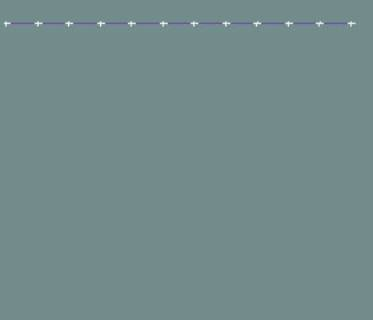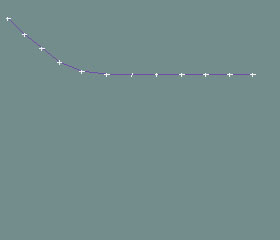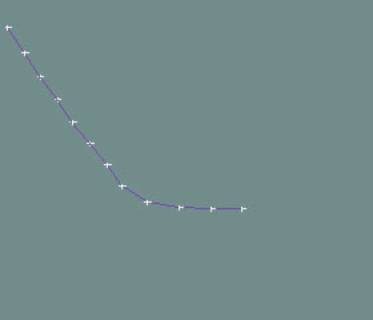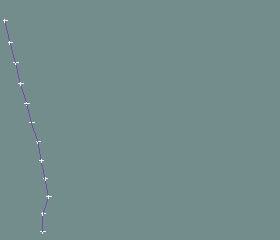Figures 9-12: Animation of the string.

Implementing Cloth and Jelly

If you understood the basic idea of the string's algorithm, implementing cloth and jelly is literally the exact same thing. You can simply use the same code you have written to simulate a string for more complex objects. The only part you need to change is the creation function (Listing 4). Then you will have to arrange the position of the nodes and the links to its neighbors to fit the cloth and jelly shapes.

As you may have notice, Listing 4 was fairly simple because a string is a simple object to setup. The cloth is not too bad to setup because it is also a very symmetric object. Yet, compared to the string, the code will be a lot more complicated. For the cloth you are going to have to setup the nodes position's as a square shape. Then, when connecting the nodes, you need to be careful with the corners, left, right, top and bottom edges and the inner parts of the cloth.

And how about the jelly? It is possible, algorithmically, to setup the jelly like a stack of cloth. Yet, the same setup code is now even more complicated than the cloth's code. First, you need to setup the node's initial position as a cubic shape. Then, when connecting the nodes you need to be careful with the corners, front, back, left, right, top and bottom planes and the inner parts of the jelly. As you can see, in this case you may have to spend a lot more time on the jelly's creation code than the physics itself. To make matters worse, if you try to model algorithmically a jelly like the one in Figure 8, the code is going to get even more tangled up. In this particular case, you are better offer to get a graphic paper, and write out the vertices positions and links and setup the node's array one by one!

Fortunately, there is a better ways to setup the cloth, jelly and more complex spring models. The main idea is to modify the creation's code for a more data driven procedure.

Data Driven Spring Models

To be able to setup the springs in a data driven way suggests, at first, the need of a tool. However, you will probably have to spend a considerable amount of time and resources to implement this tool, especially if you want to design complex 3d spring models.

Another way is to write a converter. It turns out that by simply converting geometry data from a modeler tool (3D Max, Maya, Houdini, Softimage, etc.) to spring data, you can still get good results.

The converter can be implemented by simply considering each vertex of the geometry vertex list a spring node. Then, each edge connecting two vertices becomes a spring. The basic algorithm is shown next.

for all vertices
get current vertex
for all faces
seek neighbor vertices to the current vertex
save the neighbor vertex and the "x" distance to them
loop faces
loop vertices

Although writing a converter offers several advantages, it does have a big disadvantage. Your spring model now is hooked to the geometry data, and this is not necessarily a good way to setup your springs. Most of 3D tools export geometry as a list of triangles. Therefore, your springs will always have its inner cells connected as triangles. Figure 12 depicts the jelly model arrangement converted from geometry data.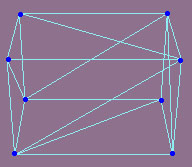Figure 12: Converted Jelly Arrangement

When perturbing and applying the spring forces to this jelly arrangement, it will morph into something unrecognizable. In other words, this arrangement is unstable. Now, if writing a tool is too much work, but converted geometry data does not work, what can you do? In a nutshell, you can do the gross arrangement of the spring model through the converter, and manually add or remove springs to fine tune your model. If you compare Figure 12 with Figure 8 you will realize that they are similar. The only difference is few inner springs connecting the bottom and top parts of the jelly.

To fine tune your springs you can write a simple tool that let you attach or delete springs from your current spring model. This tool is far less complicated than writing a spring modeler from scratch. Figure 13 shows the tool used to convert and edit the springs of the jelly in Figure 12 to the one in Figure 8.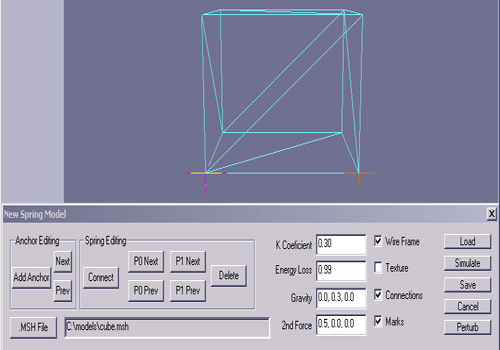Figure 13: Conversion And Editing Spring Tool

The conversion tool in figure 13 is simple mesh viewer with few extra buttons for spring editing. The interface allows the user loop through the spring nodes, add or delete springs, add anchor nodes, test the model, and tune the physics' parameters. Despite this tool interface's simplicity, it allows the user to convert, tune and test the spring model in a data driven way.

Texturing and Lighting Spring Models

So far all you have seen in this article are wireframe examples, but to texture them is trivial. When converting the geometry data to a spring model, the texture information comes with no effort. Simply move it from your original mesh data to the spring data structure. Also, you need to move the face data structure. In other words, to draw each triangle you need to know which nodes compose each face. Listing 10 illustrates the basic modifications to the main data structure to include texture information.

Listing 10: Spring Model Data Structure With Texture Information

typedef struct _spring_face
{
int i0;
int i1;
int i2; //vertex index

int t0;
int t1;
int t2; //texture index

} SPRING_FACE;

typedef struct _spring_tvert
{
float u;
float v;

} SPRING_TVERT;

typedef struct _spring
{
SPRINGM_TVERT;
SPRING_NODE node[SPRING_MAX_NODE_COUNT]; //array of spring nodes
int node_count;

SPRING_ANCHOR anchor[SPRING_MAX_ANCHOR_NODE_COUNT]; //stability & debug
int anchor_count;

SPRING_FACE* face_list; //array of face indices
int face_list_count;

SPRING_TVERT* tvert_list; //array of texture coordinates
int tvert_list_count;

float k_coef;
float energy_loss;
float mass;

} SPRING;

Figures 14, 15, 16 and 17 show a textured cloth model and four animation frames. The cloth was build by the conversion tool. Note that the red edges were deleted springs, since the original geometry does not create a good spring structure for a cloth. Four anchor points were set in a shape of a square around the center of the cloth.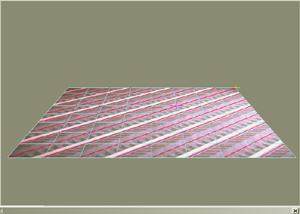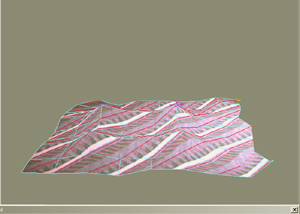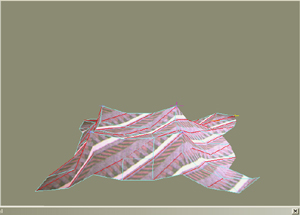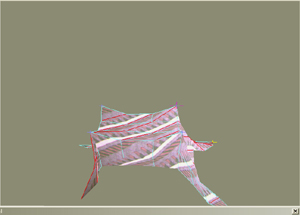Figures 14-17: Textured Cloth

Dynamic lighting is more complicated since your spring model is constantly morphing. In this case you must compute the vertex normals on the fly for each frame, and there is no way around it. The standard way is to setup pointers to neighbor faces in build time. Then, in real time, compute and average the neighbor face normals to get each vertex normal.

Complex Spring Models

With a data driven spring system, you can pretty much model and design anything you want to swing. You can build balloons, trees, tires, water, hair, tents, and so on. Even the standard applications can be enhanced, like a flag with a flexible pole for example.

Another interesting effect is to mix strings with cloth or jelly like objects. You can create a cool balloon by connecting a string to a spherical jelly. Another example would be, for a F1 game, modeling the finish line cloth with a cloth connected by strings to the poles. As the cars cross the line, apply the forces due to the air movement to the spring model.

Figure 18 shows the screen shot of this article's executable. There you will find few spring models. It has a string, a cloth, a jelly, and a balloon. Except for the string, they were modeled with the conversion tool.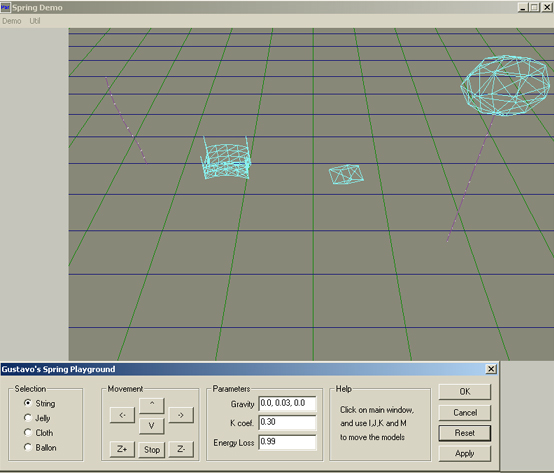Figure 18: Sample Program Screenshot

Optimizations

Spring models rely heavily on square roots, since the distance between two points is computed several times for each frame. Luckily, newer computers can handle these computations very easily for simple spring models. Also, the new consoles on the market have sets of assembler instructions to perform square roots and normalizations in single instructions by hardware. For instance, if you are a Playstation 2 developer, Listing 1 can be easily optimized using VU0 instructions.

At a higher level, the way this article's code computes the forces is redundant. If you look carefully at the code, you are always computing the same force in two opposite directions. Therefore, it makes sense to compute the force in one direction and simply negate it to get its opposite. By doing this way you are potentially cutting the inner calculations is almost half.

Sample Program

The sample program (available also at www.guitarv.com) demonstrates the standard applications (string, cloth and jelly) and a more complex object (balloon). Play around with these models and see the spring's behavior in each object. Use the interface buttons or keys I, J, K, M, Y and U move the objects. Also, the mouse (press left or right button), arrows, key A and key Z, move the camera.

The source code for the sample program is not available. Only the string implementation is available but with no render functions and no optimizations. The sample code is just to guide you on your implementation.

The more you play with springs the more ideas you have for things you can model with them. Objects modeled with springs can add more realism to your game. Yet, there are not many games out exploring this neat effect.

Next time you would like to write an interesting effect for your game, see if springs can give you new ideas. Like the famous Duke Ellington's standard says, "It Don't Mean a Thing If It Ain't Got That Swing."

Acknowledgments

I would like to thank Mattias Fagerlund from Cabrian Labs for revising the article's physics concepts.

References

 http://freespace.virgin.net/hugo.elias/
 Serway. Physics For Scientists and Enginneers, Fourth Edition, pg 364-370
 Watt, Alan, and Fabio Policarpo. The Computer Image, Addison-Wesley, 1997.
 Harris, John W., and Horst Stocker. Handbook of Mathematics and Computational Science, Springer Verlag, 1998
 Donald Hearn M. Pauline Baker. Computer Graphics, C Version, 2nd ed.
 Direct X 8 SDK Help. Microsoft Corporation
 Playstation 2 VU User's Manual, 2nd Edition. Sony Computer Entertainment Inc.

### Latest Jobs

#### Treyarch

Playa Vista, Los Angeles, CA, USA
9.12.23
Senior Level Designer (Zombies)

#### PlayStation Studios Creative Arts

Petaling Jaya, Selangor, Malaysia
9.14.23

#### Treyarch

Playa Vista, Los Angeles, CA, USA
8.22.23
Senior Gameplay Systems Engineer - Treyarch

9.18.23
VFX Artist
More Jobs

#### Game Developer Job Board

Browse open positions across the game industry or recruit new talent for your studio